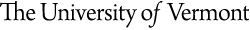#University of Vermont

|## Theoretical Condensed Matter Physics

Physics 362 Course Outline
Physics 362: Quantum Mechanics II
Course Outline

• Dirac Notation and Vector Spaces
1. Bras, kets and operators
2. Uncertainty relations
3. Postulates of quantum mechanics
• Quantum Dynamics
1. Time evolution and the Schrodinger equation
2. Schrodinger and Heisenberg pictures
3. Review of the simple harmonic oscillator
4. Path-integral approach to quantum mechanics
5. Gauge transformations and quantum mechanics
• Theory of Angular Momentum
1. Rotations and Angular Momentum
2. Spin 1/2
3. SO(3), SU(2) and Euler angles
4. Eigenvalues and eigenstates of angular momentum
5. Orbital angular momentum
7. Tensor operators
8. Wigner-Eckart theorem
• Symmetry and Quantum Mechanics
1. Symmetries, conservation laws and degeneracies
2. Group theory and quantum mechanics
• Approximation Methods
1. Time-independent perturbation theory
2. Time-dependent perturbation theory
3. Variational methods
• Identical Particles
1. Permutation symmetry
2. Many-electron systems
• Scattering Theory
1. Lippmann-Schwinger equation
2. Born approximation
3. Optical theorem
4. Applications